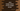# Java program to merge values of two integer arrays### Java program to merge two integer arrays :

In this Java programming tutorial, we will learn how to merge two integer arrays . The program will ask the user to enter values for the first and the second array and then it will calculate the final result by merging both the arrays. Let’s take a look at the program :

### Java program :

``````import java.util.Scanner;

public class Main {

public static void main(String[] args) {

//1
int array1_size, array2_size;

//2
int arr1[], arr2[], result[];

//3
Scanner scanner = new Scanner(System.in);

//4
System.out.print("Enter the size of the first array : ");

//5
array1_size = scanner.nextInt();

//6
System.out.print("Enter the size of the second array : ");
array2_size = scanner.nextInt();

//7
arr1 = new int[array1_size];
arr2 = new int[array2_size];
result = new int[array1_size + array2_size];

//8
System.out.println("Enter elements for the first array :");

for (int i = 0; i < array1_size; i++) {
System.out.println("Enter element " + (i + 1) + " : ");
arr1[i] = scanner.nextInt();
}

//9
System.out.println("Enter elements for the second array :");

for (int i = 0; i < array2_size; i++) {
System.out.println("Enter element " + (i + 1) + " : ");
arr2[i] = scanner.nextInt();
}

//10
for (int i = 0; i < array1_size + array2_size; i++) {
//11
if (i < array1_size) {
result[i] = arr1[i];
} else {
result[i] = arr2[i - array1_size];
}
}

//12
System.out.println("Final array after merged : ");

for (int i = 0; i < array1_size + array2_size; i++) {
System.out.print(result[i] + " ");
}
}

}
``````

### Explanation :

The commented numbers in the above program denote the step number below :

1. Create two integer variables to store the size of the first and the second array.

2. Define three integer arrays. Keep in mind that we don’t know the size of these arrays. We have not declared them yet.

3. Create one Scanner object to read the user inputs.

4. Ask the user to enter the size of the first array.

5. Read the value and store it in array1_size variable.

6. Similarly, ask the user to enter the size of the second array, read it and store it in array2_size variable.

7. Now , create the first array of size array1_size, create the second array of size array2_size and create one final array of size array1_size + array2_size.

8. Ask the user to enter the elements of the first array, using one for loop read all the numbers and store it in the first array.

9. Similarly, ask the user to enter the elements of the second array, use one for loop and store it in the second array.

10. Finally, we need to merge both of these arrays . Run one for loop. This loop will run array1_size + array2_size times.

11. Inside the loop, first put all the numbers of arr1 in the result array. Then append all the numbers of arr2 to the result array.

12. Finally, print out the merged array to the user using one for loop.

### Sample Output :

``````Enter the size of the first array : 5
Enter the size of the second array : 4
Enter elements for the first array :
Enter element 1 :
1
Enter element 2 :
2
Enter element 3 :
3
Enter element 4 :
4
Enter element 5 :
5
Enter elements for the second array :
Enter element 1 :
6
Enter element 2 :
7
Enter element 3 :
8
Enter element 4 :
9
Final array after merged :
1 2 3 4 5 6 7 8 9
``````